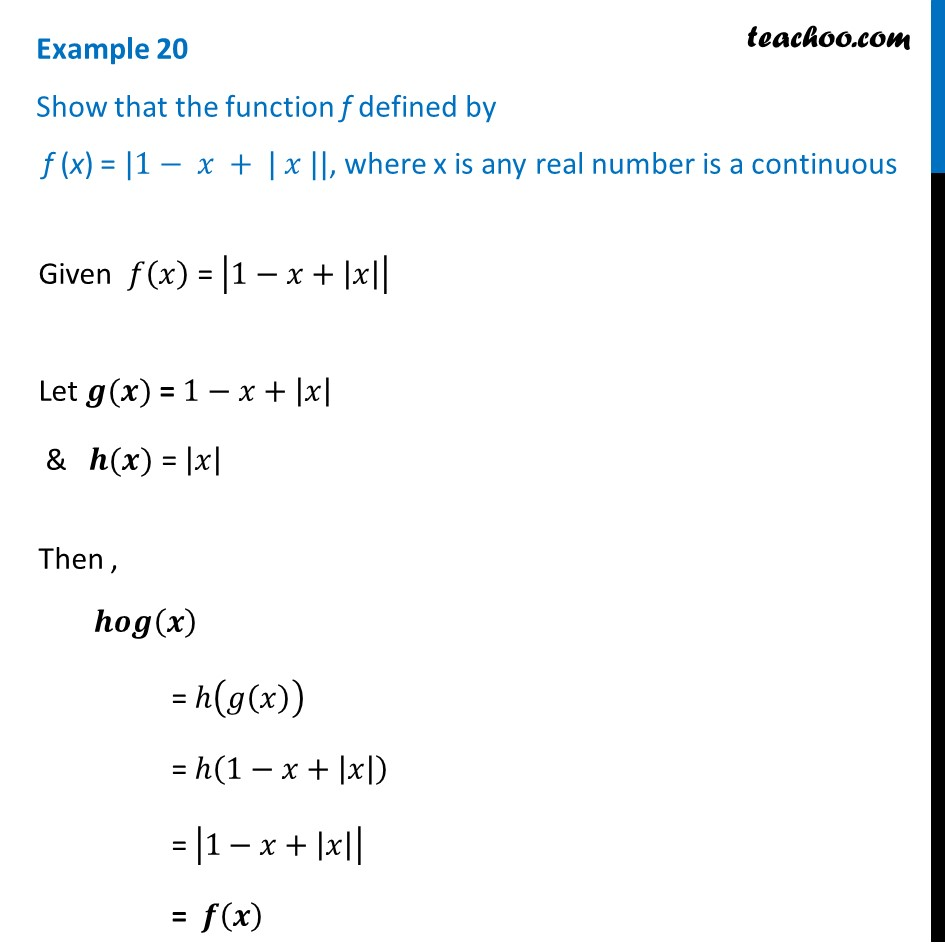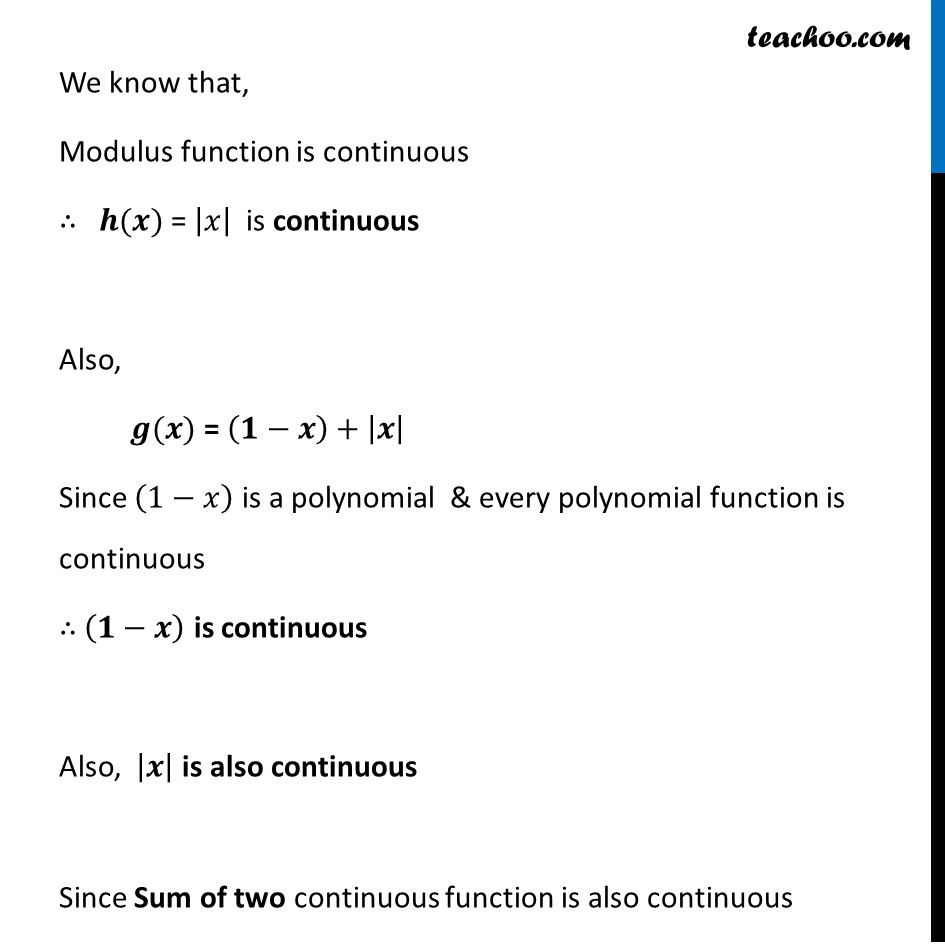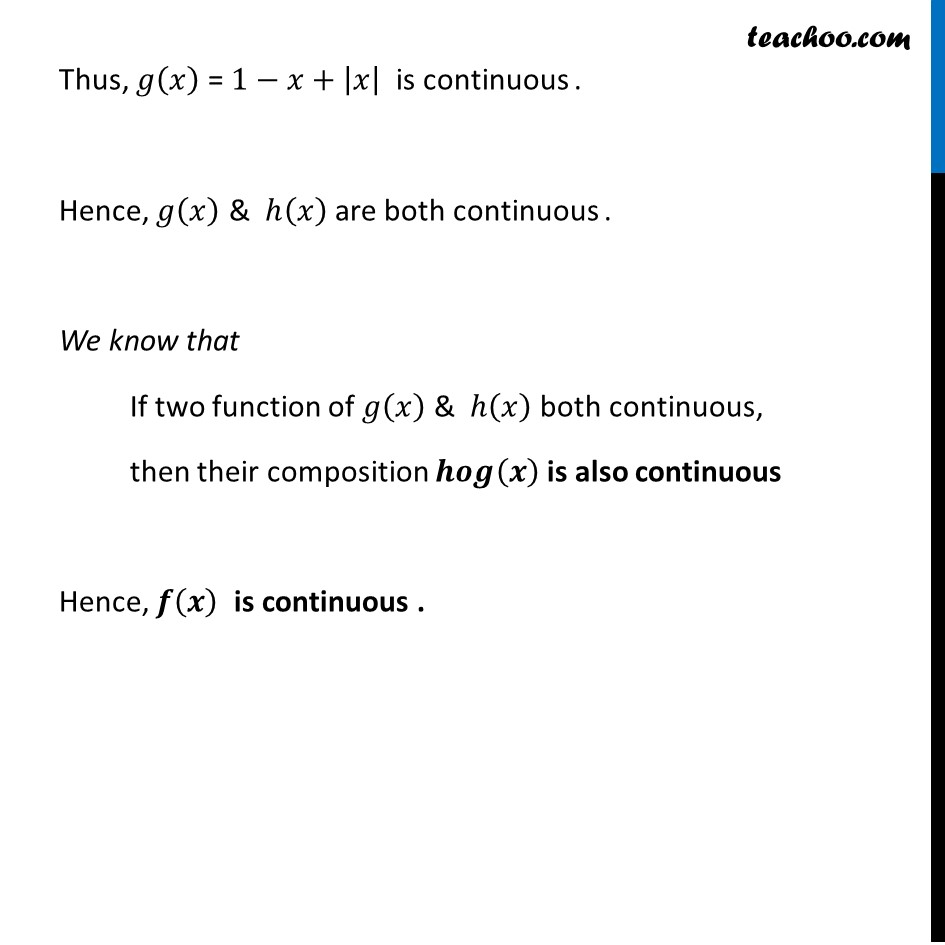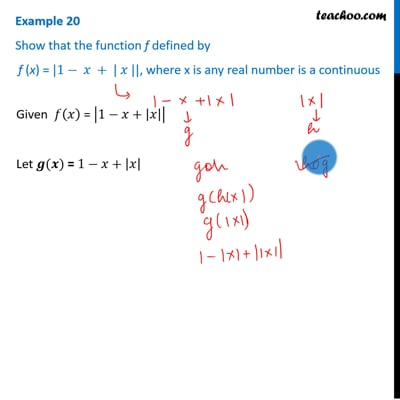Continuity of composite functions

Chapter 5 Class 12 Continuity and Differentiability
Concept wiseThis video is only available for Teachoo black users

Introducing your new favourite teacher - Teachoo Black, at only ₹83 per month

### Transcript

Example 20 Show that the function f defined by f (x) = |1− 𝑥 + | 𝑥 ||, where x is any real number is a continuousGiven 𝑓(𝑥) = |(1−𝑥+|𝑥|)| Let 𝒈(𝒙) = 1−𝑥+|𝑥| & 𝒉(𝒙) = |𝑥| Then , 𝒉𝒐𝒈(𝒙) = ℎ(𝑔(𝑥)) = ℎ(1−𝑥+|𝑥|) = |(1−𝑥+|𝑥|)| = 𝒇(𝒙) We know that, Modulus function is continuous ∴ 𝒉(𝒙) = |𝑥| is continuous Also, 𝒈(𝒙) = (𝟏−𝒙)+|𝒙| Since (1−𝑥) is a polynomial & every polynomial function is continuous ∴ (𝟏−𝒙) is continuous Also, |𝒙| is also continuous Since Sum of two continuous function is also continuous Thus, 𝑔(𝑥) = 1−𝑥+|𝑥| is continuous . Hence, 𝑔(𝑥) & ℎ(𝑥) are both continuous . We know that If two function of 𝑔(𝑥) & ℎ(𝑥) both continuous, then their composition 𝒉𝒐𝒈(𝒙) is also continuous Hence, 𝒇(𝒙) is continuous .